# Analyze the dependencies in pypi metadata¶

I parsed the requirements out of every package on pypi using the PyPi_Metatdata and Parse_requirements notebook. The output is a csv file:

• requirements.csv: Contains the packages and their formal requirements
In :
from __future__ import print_function, division

import pandas as pd
import networkx as nx
from networkx.drawing.nx_pydot import write_dot
import matplotlib.pyplot as plt
from matplotlib import patches

import seaborn as sns
import operator
import numpy as np
sns.set_context('notebook', font_scale=1.5)
sns.set_style('white')

%matplotlib inline

/home/kgullikson/anaconda3/envs/python2/lib/python2.7/site-packages/matplotlib/__init__.py:872: UserWarning: axes.color_cycle is deprecated and replaced with axes.prop_cycle; please use the latter.
warnings.warn(self.msg_depr % (key, alt_key))


## Read in the requirements data.¶

The data is stored in a csv file (separated by the '\t' character).

In :
requirements = pd.read_csv('requirements.csv')

In :
def make_graph(df, min_edges=0):
DG = nx.DiGraph()
edges = df.loc[df.requirement.notnull(), ['package_name', 'requirement']].values

DG.remove_nodes_from(['.', 'nan', np.nan])

deg = DG.degree()
to_remove = [n for n in deg if deg[n] <= min_edges]
DG.remove_nodes_from(to_remove)
return DG

In :
# Make a dotfile to import into gephi and make the network graph
DG = make_graph(requirements, min_edges=10)
write_dot(DG, 'requirements_graph.dot')

In :
dep_graph = make_graph(requirements, min_edges=0)

len(dep_graph.node)

Out:
26211

# Statistic plots¶

## 1: Histogram of the number of connections for the top several packages¶

In :
sorted_dict = sorted(dep_graph.in_degree().items(), key=operator.itemgetter(1))[::-1]

N = 10
x = np.arange(N)
y = np.array([d for d in sorted_dict[:N]])
xlabels = [d for d in sorted_dict[:N]][::-1]
fig, ax = plt.subplots(1, 1, figsize=(7, 7))

ax.barh(x[::-1], y, height=1.0)
ax.set_yticks(x + 0.5)
_ = ax.set_yticklabels(xlabels)
ax.set_xlabel('Number of Connections')
ax.set_title('Graph Degree')

fig.savefig('Figures/Connections.png')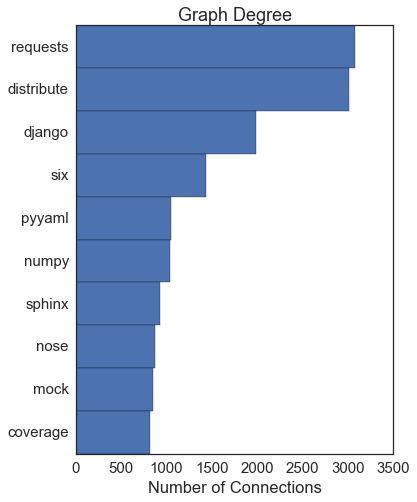## PageRank¶

Similar to the node degree, but with some extra magic.

In :
pr = nx.link_analysis.pagerank_scipy(dep_graph)
sorted_dict = sorted(pr.items(), key=operator.itemgetter(1))[::-1]

N = 10
x = np.arange(N)
y = np.array([d for d in sorted_dict[:N]])
xlabels = [d for d in sorted_dict[:N]][::-1]
xlabels = 'sphinx-py3doc-\nenhanced-theme'
fig, ax = plt.subplots(1, 1, figsize=(7, 7))

ax.barh(x[::-1], y, height=1.0)
ax.set_yticks(x + 0.5)
_ = ax.set_yticklabels(xlabels)
ax.set_xlabel('PageRank')
ax.set_title('Graph Connectivity')

fig.savefig('Figures/PageRank.png')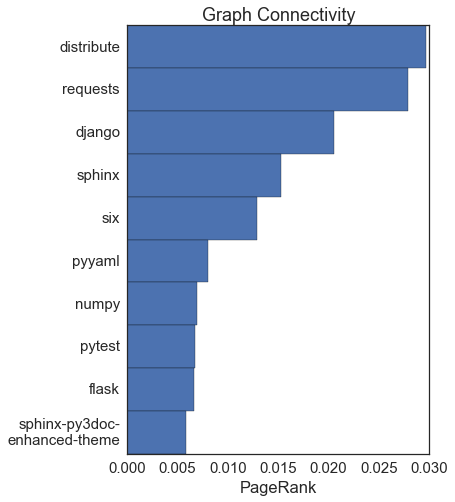# Degree Distribution¶

A random graph has a ~gaussian distribution of degrees (the number of connections to each node). Social network-type graphs are more exponential/power law with most nodes having a few connections and a few having LOTS of connections. What does this one look like?

In [ ]:
deg = dep_graph.degree()

bins=30
fig, ax = plt.subplots(1, 1, figsize=(11,4))
ax.hist(deg.values(), bins=bins, normed=False)
ax.plot(ax.get_xlim(), [1, 1], 'k--', alpha=0.5)
ax.set_xlabel('Degree')
ax.set_ylabel('Number')
ax.set_title('Degree Distribution')
ax.set_yscale('log')
ax.set_ylim((0.5, 1e5))

fig.savefig('Figures/DegreeDistribution.png')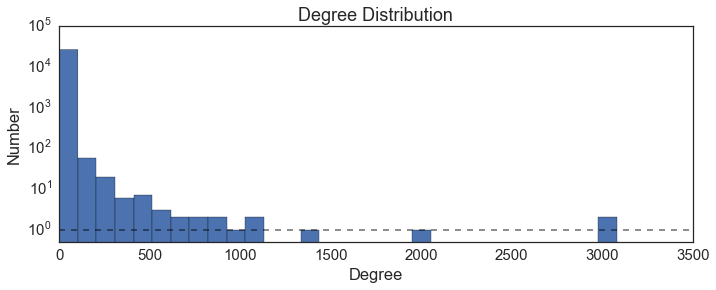Yep, this has a really fast cutoff, with the vast majority of packages having very few connections. The black line shows where there is one package in the bin. It's basically a power-law, which arises in a "rich get richer" model. In other words, some packages (the ones with very high degree) are required by many things, so new packages also require them. They also tend to be older packages, which means they've had longer to accumulate packages that depend on them.

# Centrality Measures¶

The statistics above are basically degree centrality measures, but there are actual statistics that look at it too.

• Betweenness Centrality The betweenness centrality of node n is defined as the proportion of best paths between any other pairs of nodes which pass through n.
In [ ]:
bc = nx.betweenness_centrality(dep_graph)

In :
sorted_dict = sorted(bc.items(), key=operator.itemgetter(1))[::-1]

N = 10
x = np.arange(N)
y = np.array([d*100 for d in sorted_dict[:N]])
xlabels = [d for d in sorted_dict[:N]][::-1]
fig, ax = plt.subplots(1, 1, figsize=(7, 7))

ax.barh(x[::-1], y, height=1.0)
ax.set_yticks(x + 0.5)
_ = ax.set_yticklabels(xlabels)
ax.set_xlabel('Betweenness Connectivity (%)')

fig.savefig('Figures/Betweenness.png')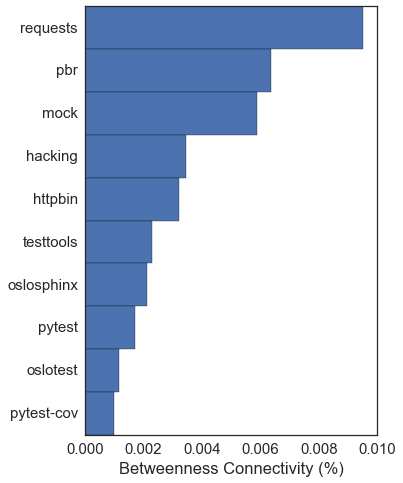# Is this a small-world graph?¶

Compute both the average path length and the clustering coefficient. Small worlds have large clustering coefficients and small average path lengths.

In :
avg_pathlen = []
subgraph_size = []
for subgraph in nx.connected_component_subgraphs(dep_graph.to_undirected()):
n = len(subgraph)
if n > 1:
subgraph_size.append(n)
avg_pathlen.append(nx.average_shortest_path_length(subgraph))

avg_clustering = nx.average_clustering(dep_graph.to_undirected())
apl = np.average(avg_pathlen, weights=subgraph_size)
print(apl, avg_clustering)

4.12485074254 0.0687035243533


So I'm not sure... by the definition above, the clustering coefficient seems to be quite small. That means that node neighborhoods are not connected anywhere near as much as they could be, and that this is NOT a small-world graph. On the other hand, the average path length $L \sim \log{N}$, which is another definition of a small-world graph. I guess these could be reconciled if there are a few very very important nodes that act as hubs, but the "spokes" that come out of the hubs are relatively independent. Those hubs appear to be requests and django, and maybe six.

# Matrix Graph¶

(code taken from the StackOverflow)

The nodes are partitioned using the community code, which separates a large graph into communities as follows:

2. For each node, test if the "modularity" increases by putting it into the same community as one of it's neighbors (one of the nodes it is connected to)
3. Continue until no more gain is found
4. Make a NEW network where each node is now the communities found in the above set.
5. Repeat steps 1-3 many times, until the modularity no longer increases.

Details on the algorithm can be found here. You might notice that this algorithm is basically creating a dendrogram of the network.

In :
from scipy.sparse import coo_matrix

def plot_coo_matrix(m, partitions=None, colors=None):
if not isinstance(m, coo_matrix):
m = coo_matrix(m)

fig = plt.figure(figsize=(9, 9))
ax.plot(m.col, m.row, 's', color='black', ms=1)
ax.set_xlim(0, m.shape)
ax.set_ylim(0, m.shape)
ax.set_aspect('equal')
for spine in ax.spines.values():
spine.set_visible(False)
ax.invert_yaxis()
ax.set_aspect('equal')
ax.set_xticks([])
ax.set_yticks([])

# The rest is just if you have sorted nodes by a partition and want to
# highlight the module boundaries
if partitions is None and colors is None:
return

assert len(partitions) == len(colors)
for partition, color in zip(partitions, colors):
current_idx = 0
for module in partition:
len(module), # Width
len(module), # Height
facecolor="none",
edgecolor=color,
linewidth="1"))
current_idx += len(module)
return

def find_closest(G, node, node_list):
""" Find which node in node_list the given node is closest to
"""
min_pathlen = np.inf
best_node = "Isolated"
for n in node_list:
if nx.has_path(G, node, n):
length = nx.shortest_path_length(G, node, n)
if length < min_pathlen:
min_pathlen = length
best_node = n
return best_node

In :
import community

#first compute the best partition
partition = community.best_partition(dep_graph.to_undirected())

In :
from collections import defaultdict

louvain_comms = defaultdict(list)
for node_index, comm_id in partition.iteritems():
louvain_comms[comm_id].append(node_index)
louvain_comms = louvain_comms.values()

nodes_ordered = [node for comm in louvain_comms for node in comm]

plot_coo_matrix(matrix)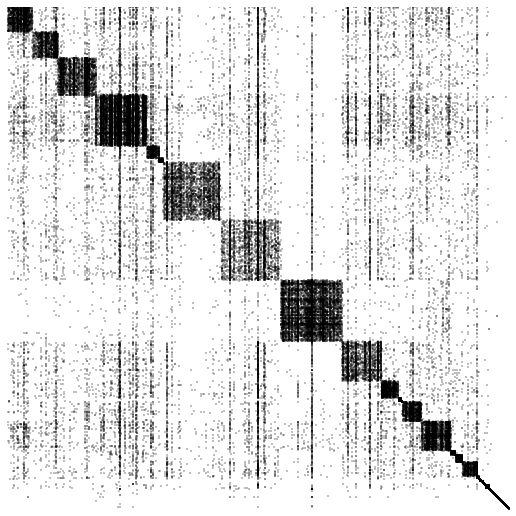## What do the communities signify?¶

I will try to find the significance of the 8 large communities shown in the figure above.

In :
# Get the size of each community
comm_size = {i: len(louvain_comms[i]) for i in range(len(louvain_comms))}

# Sort by size
big_community_sizes = sorted(comm_size.items(), key=operator.itemgetter(1), reverse=True)[:10]

# Get the packages in each of the communities
big_communities = [louvain_comms[i] for i in sorted([c for c, s in big_community_sizes])]

In :
def plot_community(i, N=5):
# Make a subgraph
subgraph = dep_graph.subgraph(big_communities[i])

# Find the most important nodes in the subgraph:
sorted_dict = sorted(pr.items(), key=operator.itemgetter(1), reverse=True)

N = 5
x = np.arange(N)
y = np.array([d for d in sorted_dict[:N]])
xlabels = [d for d in sorted_dict[:N]][::-1]
fig, (left, right) = plt.subplots(1, 2, figsize=(13, 7))

left.barh(x[::-1], y, height=1.0)
left.set_yticks(x + 0.5)
_ = left.set_yticklabels(xlabels)
left.set_xlabel('PageRank')
left.set_title('Graph Connectivity')

alpha = 200.0/len(subgraph.nodes())
print(alpha)
nx.draw(subgraph, ax=right, node_size=10, alpha=alpha)

In :
# Flask and web framework development
plot_community(i=0)

0.151515151515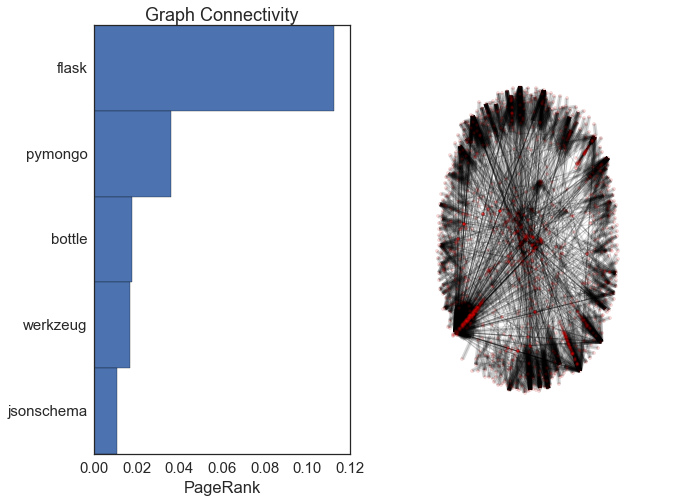In :
# Not sure. redis is a data store, and tornado is a kind of web server.
#tornado and pyzmq get used in jupyter notebook, but that is not in this community...
plot_community(i=1)

0.14847809948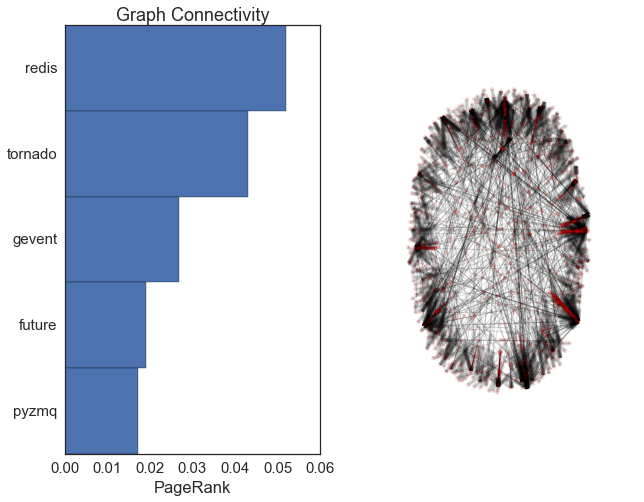In :
#pydata
plot_community(i=2)

0.102354145343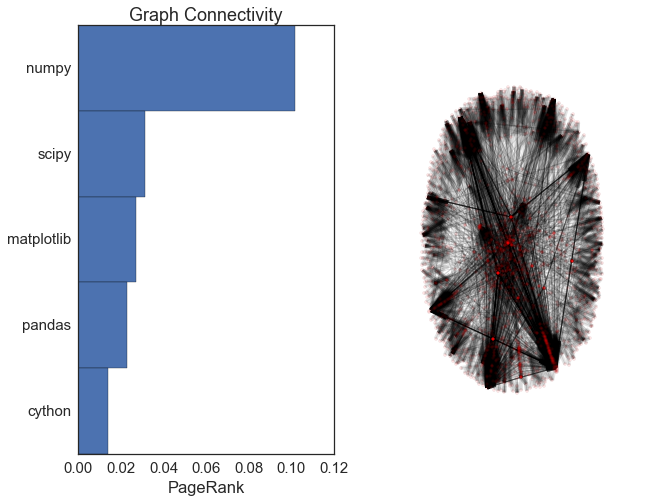In :
# Testing and documentation
plot_community(i=3)

0.0752445447705